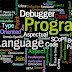## NECESSARY PARAMETER IN PROGRAMING

Most high level programing language are Object oriented program (OOP). An OOP is use as one of the ways to access or call to action the operations of a class.

Example in a class JSS1, the objects of the class can be the teacher, students, white board, maker, desk etc. Also in a class student, the object of that class are school uniform, bag, writing materials, mathematical set, calculator etc.

Editors this allows the user to enter the program source code and save it to files. Most programming editors increase programmer productivity by using colors to highlight language features.

Subroutine this is a section of code that is called to the main body of a program by mean of object to implement a task. It may be used in more than one point in the program.

### ALGORITHM USING PSEUDO CODE

Problem 1: write an algorithm to add two numbers and print the result on the screen.

Step 1: Start
Step 2: double num1, num2, sum
Step 3: Enter num1 and num2
Step 4: sum = num1 + num2
Step 5: Print sum
Step 6: Stop

Assignment 1: Write an algorithm to calculate the factorial for a given number entered from standard keyboard.

Step 1: Start
Step 2: Integer Number, factorial = 1, test = 0 (Declaration)
Step 3: Enter Number (Prompt the user)
Step 4: factorial = factorial * (Number - test)
Step 5: If (Number = 0) Print factorial = 1
Step 6: If (Number > Test), Do Test = Test + 1 and Goto Step4
Step 7: Print “the factorial = ” factorial
Step 8: Stop

Assignment 2: write an algorithm to enter number from the keyboard and determine if the number is prime or not.

Step 1: Start
Step 2: Declaration integer number, A = 2, B = 3
Step 3: Enter number
Step 4: If (number = A and number = B)) then Print ‘’ the number is PRIME’’
Step 5: if (number%2>0) or (number%3>0) print “the number is PRIME”
Step 6: If (number%2=0) or (number%3=0) then Print ‘’the number is not PRIME’’
Step 7: stop

Assignment 3: write an algorithm to determine the root of a quadratic equation.

Step 1: Start
Step 2: double a, b, c, x1, x2, and d
Step 3: Enter a, b, c
Step 4: sqrt (b^2-4*a*c)
Step 5: if (d>0), x1 = (-b+d)/2*a and x2 = (-b-d)/2*a
Step 6: if (d=0), x1 = x2 = -b/2*a Step7: if (d<0), x1 = “complex number”
Step 8: print x1 and x2
Step 9: stop

Problem 2: write an algorithm to find the largest value of 3 numbers entered from the keyboard.

Step 1: Start
Step 2: double A, B, and C
Step 3: Enter A, B, and C
Step 4: If (A > B) and (A > C) then Print ‘’ the Max = A’’
Step 5: If (B > A) and (B > C) then Print ‘’ the Max = B’’
Step 6: If (C > A) and (C > B) then Print ‘’ the Max = C’’
Step 7: Stop

Problem 4: write an algorithm to calculate the average of 50 scores

Step 1: Start
Step 2: double score, sum = 0, counter = 1, average
Step 3: Enter score
Step 4: While (counter <= 50), sum = sum + score, counter = counter +1. Goto step 3
Step 5: = average = sum/50.0
Step 6: Print “Average =” average.
Step 7: Stop

Problem 5): Design an algorithm to input the radius of a circle and calculate the circumference and area and output the values.

Step 1: Start
Step 2: double Radius, Circumference, Area, and PI = 3.142
Step 3: Enter Radius
Step 4: Circumference = 2*PI* Radius
Step 5: Area = PI* Radius* Radius
Step 6: Print Circumference and Area
Step 7: Stop

Problem 6: Develop an algorithm that will prompts the user for 3 resistance values and output the result in parallel.

Step 1: Start
Step 2: double R1, R2, R3, Rt, and Rs
Step 3: Enter R1, R2 and R3
Step 4: Rt = R1*R2*R3
Step 5: Rs = (R2*R3) + (R1*R3) + (R1*R2)
Step 6: Rparallel = Rt/Rs
Step 7: Print Rparallel
Step 8: Stop

### ALGORITHM USING FLOW CHARTS

This is a visual representation of sequential steps in solving a particular problem. It involves the connections different shapes using directional arrows to indicate the flow pattern.

The basic symbols commonly used in flow charting of assembly language programs are not limited to the following:

Terminal, Process, Input/Output, Decision, Connector and Predefined process.

### SYMBOL NAME FUNCTION

Processing: Indicates any types of internal operation and computation inside the processor or Memory.

Predefined process: Used to invoke a subroutine or an Interrupt program.

Input/output: Used for any input / output (I/O) operation. Indicates that the computer is to obtain and display data.

Decision: Used to ask a question that can be answered in a binary format (Yes/No, True/False)

Terminal: Indicates the starting or stopping of the program, progress, or interrupt program.

Connection: Allows the flowchart to be drawn without a reserve flow. It serve as a link and continuation.

Flow lines: Shoe direction of flow.

### ORDER OF OPERATIONS

The operations in computer programming follows this pattern of Bracket, Exponential, Division, Multiplication, Addition, and Subtraction (BEDMAS).

It is not the executable code, but the structure of BEDMAS is peculiar different programing language but fore thee general view I will take the exponential as (**)

Examples are;

Answer = 3 + 5 * 4 = 23
Answer = (3 + 5) * 4 = 23
Answer = 3**2 / 3 * 4 + 6/2 = 15
Answer = 3**2/3 * (4 + 6)/2 = 15.

It produce the same result but the processing is different.

Introduction To Programming (Two) 2: Computer Programming For BeginnersReviewed by Chukwumah Rapheal on March 09, 2016 Rating: 5